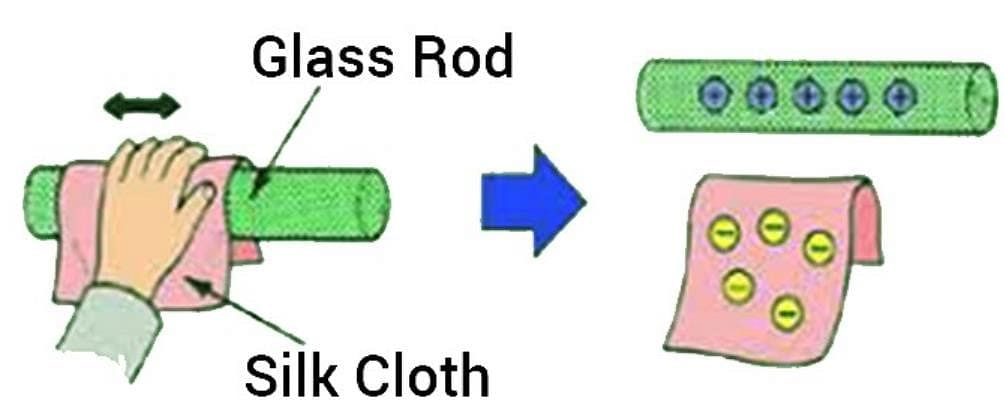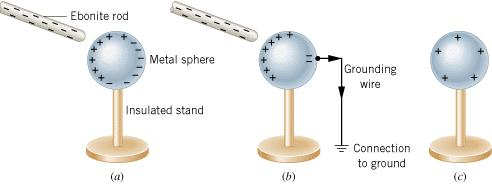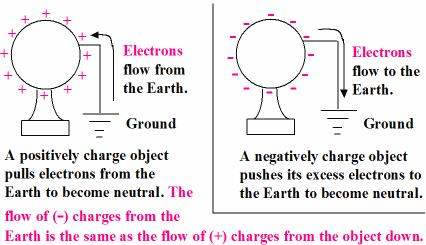NEET  >  Test: Electric Charge

# Test: Electric Charge

Test Description

## 20 Questions MCQ Test | Test: Electric Charge

Test: Electric Charge for NEET 2023 is part of NEET preparation. The Test: Electric Charge questions and answers have been prepared according to the NEET exam syllabus.The Test: Electric Charge MCQs are made for NEET 2023 Exam. Find important definitions, questions, notes, meanings, examples, exercises, MCQs and online tests for Test: Electric Charge below.
Solutions of Test: Electric Charge questions in English are available as part of our course for NEET & Test: Electric Charge solutions in Hindi for NEET course. Download more important topics, notes, lectures and mock test series for NEET Exam by signing up for free. Attempt Test: Electric Charge | 20 questions in 20 minutes | Mock test for NEET preparation | Free important questions MCQ to study for NEET Exam | Download free PDF with solutions
 1 Crore+ students have signed up on EduRev. Have you?
Test: Electric Charge - Question 1

### A material in which electrons are tightly bound and cannot move freely at room temperature is called _________.

Detailed Solution for Test: Electric Charge - Question 1

Insulators are materials that impede the free flow of electrons from atom to atom and molecule to molecule. Examples of insulators include plastics, paper, rubber, glass and dry air.

In contrast to insulators, Conductors are materials that permit electrons to flow freely from particle to particle. Examples of conductors include metals, aqueous solutions of salts (i.e., ionic compounds dissolved in water), graphite, and the human body.

Test: Electric Charge - Question 2

### According to the principle of conservation of charge, in closed system:

Detailed Solution for Test: Electric Charge - Question 2
• The law of conservation of charge states that electric charge can neither be created nor destroyed.
• In a closed system, the amount of charge remains the same. When something changes its charge, it doesn't create a charge but transfers it.

Therefore, the correct answer is the algebraic sum of charges in any closed system is constant.

Test: Electric Charge - Question 3

### A coulomb is the same as:​

Detailed Solution for Test: Electric Charge - Question 3

We know that Current = Charge / Time

Also,

Current = Ampere

Charge = Coulomb

Time = Second

1 ampere = 1 coulomb/ 1 sec

So, one coulomb is ampere times second.

Test: Electric Charge - Question 4

When a glass rod is rubbed with silk, it becomes positively charged because:

Detailed Solution for Test: Electric Charge - Question 4

When the glass rod is rubbed with silk cloth, glass quickly loses electrons, and silk takes electrons out of the glass rod. So after rubbing, the glass rod becomes positively charged and the silk gets a negative charge.

Silk cloths: Negatively charged

Glass rod: Positively chargedTest: Electric Charge - Question 5

Electric charge between two bodies can be produced by:

Detailed Solution for Test: Electric Charge - Question 5
• Electric charge between two bodies can be produced by rubbing with each other. This is known as triboelectric effect.
• The body having lower work function loses electron and becomes positive and the other body gains electrons become negative.

Example: When glass is rubbed with silk, glass becomes positive while silk becomes negative.

Test: Electric Charge - Question 6

Which of the following methods can be used to charge a metal sphere positively without touching it? Choose the best possible option:

Detailed Solution for Test: Electric Charge - Question 6

This can be possible by induction, if a negative charge rod is placed near the sphere (at a distance not in touch) then it attracts positive charge inside the sphere towards its side and the negative charges collected on the other side and when the surface of the sphere connected to the earth than the negative charge goes to earth and the sphere remain positively charged.Since rubbing the metal ball with fur and a piece of silk cloth involves touching, they can not be the correct answer.

Therefore, the correct answer is C.

Test: Electric Charge - Question 7

If an object is positively charged, theoretically the mass of the object ______.

Detailed Solution for Test: Electric Charge - Question 7
• If an object is positively charged it means it has lost some of its electrons.
• The mass of an electron is 9.11*10-31 kg.
• So, if a positively charged body loses the ‘n’ number of electrons, its mass decreases by the amount n*9.11*10-31kg.
Test: Electric Charge - Question 8

Choose the correct statement:

Detailed Solution for Test: Electric Charge - Question 8

According to the principle of conservation of charge, the net amount of positive charge minus the net amount of negative charge in the universe is always constant.

Thus, the correct answer is the total charge of the universe is constant.

Test: Electric Charge - Question 9

To make an uncharged object to have a negative charge we must:

Detailed Solution for Test: Electric Charge - Question 9

To make an uncharged object have a negative charge we must increase negative charge particles in it and this can be done only by adding electrons to particles as electrons have negative charge.

Test: Electric Charge - Question 10

When conductors are placed in an electric field, their electrons are moved:

Detailed Solution for Test: Electric Charge - Question 10
• A substance is said to be a conductor if it conducts electricity.
• When placed in an electric field, the electrons move in the opposite direction of the current, so it is able to conduct.

Explanation:

• A charged conductor is placed in an external electric field.
• The charge is distributed on the surface of the conductor.
• The free electrons move opposite the direction of the external electric field.
• Thus more charges are induced on the surface of the conductor due to this movement of free electrons.
• The free electrons move until the force due to the external field is negated completely due to the force by induced charges.
• At this point, the net force on a free electron inside the conductor is zero.
• Hence, making the net electric field inside the conductor is zero.
Test: Electric Charge - Question 11

When a negatively charged conductor is connected to earth,

Detailed Solution for Test: Electric Charge - Question 11
• When a negatively charged body is grounded electrons from the body flow to the earth and the body becomes neutral.
• On the other hand, when a positively charged body is grounded electrons coming from the earth neutralize the body.Additional Information: Earth is an electric conductor. Earthing is defined as “the process in which the instantaneous discharge of the electrical energy takes place by transferring charges directly to the earth through low resistance wire.” When a charged body is connected to the earth, it becomes electrically neutral.

Test: Electric Charge - Question 12

A negative ion is an atom that ________ electrons.

Detailed Solution for Test: Electric Charge - Question 12
• Ions are formed when atoms gain or lose electrons.
• Gaining electrons changes an atom into a negative ion.
• Losing electrons changes an atom into a positive ion.

Therefore, the correct answer is C.

Test: Electric Charge - Question 13

When a hard rubber rod is given a negative charge by rubbing it with wool:

Detailed Solution for Test: Electric Charge - Question 13
• On rubbing a rubber rod with wool, the electrons from the wool get transferred to the rubber rod.
• The wool now has a deficiency of electrons and so is positively charged.
• On the other hand, the rubber rod has an excess of electrons and hence is negatively charged.
• In the above example, the wool undergoes positive electrification.
• It is to be noted that it is the electrons and not the protons that get transferred during positive (and negative) electrification.
• Here, the transfer of electrons and mass transfer respectively takes place from wool to the rubber rod.
Test: Electric Charge - Question 14

The value of charge on a body which carries 30 excess electrons is:

Detailed Solution for Test: Electric Charge - Question 14

Here, n = 30
e = -1.6 x 10-19 C
We know that q = n ∗ e

Therefore, q = 30 * (1.6 x 10-19 C)
q = - 4.8 ∗ 10-18 C

Test: Electric Charge - Question 15

The total negative charge in 1 mol of helium (atomic number 2, atomic mass 4) is:

Detailed Solution for Test: Electric Charge - Question 15
• He atom has 2 electrons.
• So, 1 mole of He has 2*N(N is Avogadro's no.) electrons.
• Then total -ve charge in 1 mole He gas is =  2 * N * charge of 1 electron
= 2 * (6.022 * 1023) * (1.6 * 10-19) = 1.92 * 105
Test: Electric Charge - Question 16

Name the physical quantity whose S.I. unit is JC-1. Is it a scalar or a vector quantity?

Detailed Solution for Test: Electric Charge - Question 16
• Physical quantity whose S.I. unit is JC-1 is Electric potential.
• It is a Scalar quantity.

• The SI base unit of electric flux is voltmeters (V m) which is also equal to newton-meters squared per coulomb (N m2 C-1). Also, electric flux is a scalar quantity.
• Electric potential is expressed in units of joules per coulomb (i.e., volts). It is also a scalar quantity.

Test: Electric Charge - Question 17

How many electrons are present in 1 coulomb of charge?​

Detailed Solution for Test: Electric Charge - Question 17

According to Quantisation of Charge we know that,
Q = ne
Therefore, 1 coulomb of Charge = n * 1.6 * 10-19
⇒ 1/ 1.6 × 10-19 = n
⇒ n = 6.25 × 1018

Test: Electric Charge - Question 18

What does q1 + q2 = 0 signify?​

Detailed Solution for Test: Electric Charge - Question 18

If we have two bodies with charge q1 and q2 on them and we are given q1 + q2 = 0. It means the net charge on the system is zero.

This is possible only if q1 and q2 are equal and opposite.

That is, q1 = +q and q2 = -q or vice versa.

Such that, q1 + q2 = +q + (-q) = 0

Test: Electric Charge - Question 19

A silk cloth rubbed with a glass rod acquire a charge (-1.6 x 10-19) C. Then the charge on the glass rod is​:

Detailed Solution for Test: Electric Charge - Question 19

During rubbing, glass rod acquires + ve charges and silk cloth acquires - ve charges.
The glass rod will have postive charge equal in value to the negative charge lost by the silk.
∴ the charge on the glass rod is + 1.6×10- 19C

Additional Information: When a glass rod is rubbed with silk, glass happens to lose electrons easily and silk grabs them away from the glass atoms. So after rubbing, the glass becomes positively charged and the silk becomes negatively charged. Thus equal but opposite charges are produced on both.

Test: Electric Charge - Question 20

To make uncharged object have a positive charge:

Detailed Solution for Test: Electric Charge - Question 20
• A body consists of atoms that have electrons, protons and neutrons. The electrons have a negative charge. The protons are positively charged and the neutrons are neutral.
• The atom is neutral because the positive and negative charges cancel out each other.
• On removing electrons, an excess of protons are present and thus the body is said to be positively charged.
 Use Code STAYHOME200 and get INR 200 additional OFF Use Coupon Code
Information about Test: Electric Charge Page
In this test you can find the Exam questions for Test: Electric Charge solved & explained in the simplest way possible. Besides giving Questions and answers for Test: Electric Charge, EduRev gives you an ample number of Online tests for practice

### How to Prepare for NEET

Read our guide to prepare for NEET which is created by Toppers & the best Teachers